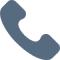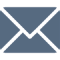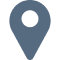# Geometry

Please note that RMXplore's Math Bytes Courses are not
currently open for registration
.

### Geometry

Coming Soon!
Course Pricing:
• Online : \$260
• Houston Center : \$320
• Cupertino Center : \$400
Course Logistics:
Duration : to be updated
Number of Classes: 5 weeks course
Occurs : to be updated

### Math Byte Course Topics:

Course Topics are organized as six modules with five granular topics covered under each module. Click through below to see all modules/topics for this course.

#### 01 - Introduction to Geometry• Angles in a Triangle
• Congruent Triangles
• Isosceles and Equilateral Triangles
• Similar Triangles
• Area and Perimeter

#### 02 - Basic Properties of a Triangle• Angles in a Triangle
• Congruent Triangles
• Isosceles and Equilateral Triangles
• Similar Triangles
• Area and Perimeter

#### 03 - Special Properties of a Triangle• Right Triangles
• Angle Bisector Theorem & Incenter
• Perpendicular Bisectors and Circumcenter
• Altitudes and Orthocenter
• Medians and Centroids

#### 04 - Quadrilaterals and Polygons• Parallelograms, Rectangles and Squares
• Trapezoids and Rhombus
• Pentagon, Hexagon, and Octagon
• Special Topics and Theorems

#### 05 - Circles• Angles - Inscribed and Central
• Circular Segments and Arc Length
• Sectors and Area
• Properties of Tangents
• Power of a Point

#### 06 - Special Topics in Geometry• Angles - Inscribed and Central
• Circular Segments and Arc Length
• Sectors and Area
• Properties of Tangents
• Power of a Point